## WebAssembly 学习笔记

WebAssembly（简称 WASM）是一种以安全有效的方式运行可移植程序的新兴 Web 技术，下面是引用 MDN 上对它的定义

WebAssembly 是一种新的编码方式，可以在现代的网络浏览器中运行 － 它是一种低级的类汇编语言，具有紧凑的二进制格式，可以接近原生的性能运行，并为诸如 C/C++ 等语言提供一个编译目标，以便它们可以在 Web 上运行。它也被设计为可以与 JavaScript 共存，允许两者一起工作。WebAssembly 在 2019 年 12 月 5 日被万维网联盟（W3C）推荐为标准，与 HTML，CSS 和 JavaScript 一起，成为 Web 的第四种语言。

## WebAssembly 之前的历史

JavaScript 诞生于 1995 年 5 月，一个让人津津乐道的故事是，当时刚加入网景的 Brendan Eich 仅仅花了十天时间就开发出了 JavaScript 语言。开发 JavaScript 的初衷是为 HTML 提供一种脚本语言使得网页变得更动态，当时根本就没有考虑什么浏览器兼容性、安全性、移植性这些东西，对性能也没有特别的要求。但随着 Web 技术的发展，网页要解决的问题已经远不止简单的文本信息，而是包括了更多的高性能图像处理和 3D 渲染等方面，这时，JavaScript 的性能问题就凸显出来了。于是，如何让 JavaScript 执行的更快，变成了各大浏览器生产商争相竞逐的目标。

### 浏览器性能之战

JIT 技术推出之后，JavaScript 的性能得到了飞速提升：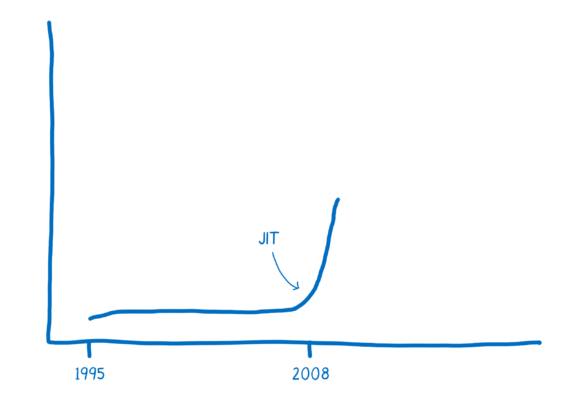``````function arraySum(arr) {
var sum = 0;
for (var i = 0; i < arr.length; i++) {
sum += arr[i];
}
}``````

``arr = [1, "hello"];``

JavaScript 这种动态类型的特点对 JIT 引擎是非常不友好的，反复的优化和去优化不仅无法提高性能，甚至会有副作用。所以在实际的生产环境中，JIT 的效果往往没有那么显著，通过 JIT 的优化很快就遇到了瓶颈。

Google 在 2008 年开源了 NaCl 技术，并在 2011 年的 Chrome 14 中正式启用。NaCl 的全称为 Native Client，这是一种可以在浏览器中执行原生代码（native code）的技术，听起来很像是 Microsoft 当时所使用的 ActiveX 技术，不过 ActiveX 由于其安全性一直被人所诟病。而 NaCl 定义了一套原生代码的安全子集，执行于独立的沙盒环境之中，并通过一套被称为 PPAPI（Pepper Plugin API）的接口来和 JavaScript 交互，避免了可能的安全问题。NaCl 采取了和 JIT 截然不同的 AOT 编译（Ahead-of-time compilation，即提前编译）技术，所以在性能上的表现非常突出，几乎达到了和原生应用一样的性能。不过由于 NaCl 应用是 C/C++ 语言编写的，与 CPU 架构强关联，不具有可移植性，因此需要针对不同的平台进行开发以及编译，用户使用起来非常痛苦。

### Mozilla 的 asm.js 解决方案

2010 年，刚刚加入 Mozilla 的 Alon Zakai 在工作之余突发奇想，能不能将自己编写的 C/C++ 游戏引擎运行在浏览器上？当时 NaCl 技术还没怎么普及，Alon Zakai 一时之间并没有找到什么好的技术方案。好在 C/C++ 是强类型语言，JavaScript 是弱类型语言，所以将 C/C++ 代码转换为 JavaScript 在技术上是完全可行的。Alon Zakai 于是便开始着手编写这样的一个编译器，Emscripten 便由此诞生了！

Emscripten 和传统的编译器很类似，都是将某种语言转换为另一种语言形式，不过他们之间有着本质的区别。传统的编译器是将一种语言编译成某种 low-level 的语言，比如将 C/C++ 代码编译成二进制文件（机器码），这种编译器被称为 Compiler；而 Emscripten 是将 C/C++ 代码编译成和它 same-level 的 JavaScript 代码，这种编译器被称为 Transpiler 或者 Source to source compiler

Emscripten 相比于 NaCl 来说兼容性更好，于是很快就得到了 Mozilla 的认可。之后 Alon Zakai 被邀请加入 Mozilla 的研究团队并全职负责 Emscripten 的开发，以及通过 Emscripten 编译生成的 JavaScript 代码的性能优化上。在 2013 年，Alon Zakai 联合 Luke Wagner，David Herman 一起发布了 asm.js 规范，同年，Mozilla 也发布了 Firefox 22，并内置了新一代的 OdinMonkey 引擎，它是第一个支持 asm.js 规范的 JavaScript 引擎。

asm.js 的思想很简单，就是尽可能的在 JavaScript 中使用类型明确的参数，并通过 `TypedArray` 取消了垃圾回收机制，这样可以让 JIT 充分利用和优化，进而提高 JavaScript 的执行性能。比如下面这样一段 C 代码：

``````int f(int i) {
return i + 1;
}``````

``````function f(i) {
i = i|0;
return (i + 1)|0;
}``````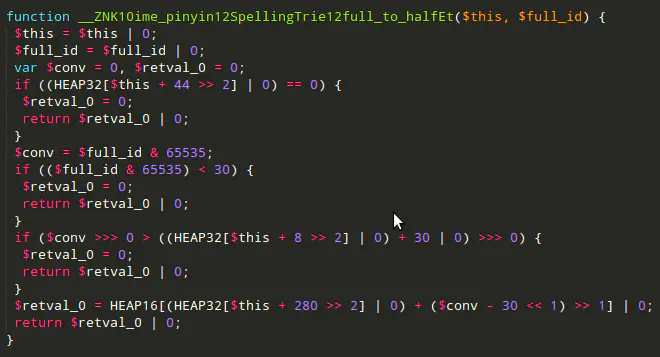### WebAssembly = NaCl + asm.js

• 和 NaCl/PNaCl 一样，基于二进制格式，从而能够被快速解析，达到原生代码的运行速度；
• 和 PNaCl 一样，依赖于通用的 LLVM IR，这样既具备可移植性，又便于其他语言快速接入；
• 和 asm.js 一样，使用 Emscripten 等工具链进行编译；另外，Emscripten 同时支持生成 asm.js 和二进制格式，当浏览器不兼容新的二进制格式时，asm.js 可以作为降级方案；
• 和 asm.js 一样，必须以非常自然的方式直接操作 Web API，而不用像 PNaCl 一样需要处理与 JavaScript 之间的通信；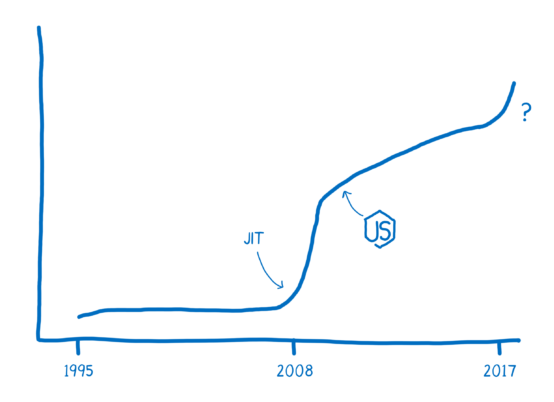## WebAssembly 入门示例

### 将 C/C++ 程序编译成 WebAssembly

``````\$ emcc --check
shared:INFO: (Emscripten: Running sanity checks)``````

``````#include <stdio.h>

int main() {
printf("Hello World\n");
return 0;
}``````

``\$ emcc hello.c -o hello.html``

• hello.wasm - 这就是生成的 WebAssembly 二进制字节码文件
• hello.js - 包含一段胶水代码（glue code）通过 JavaScript 来调用 WebAssembly 文件
• hello.html - 方便开发调试，在页面上显示 WebAssembly 的调用结果

``\$ npx serve .``

``\$ python3 -m http.server``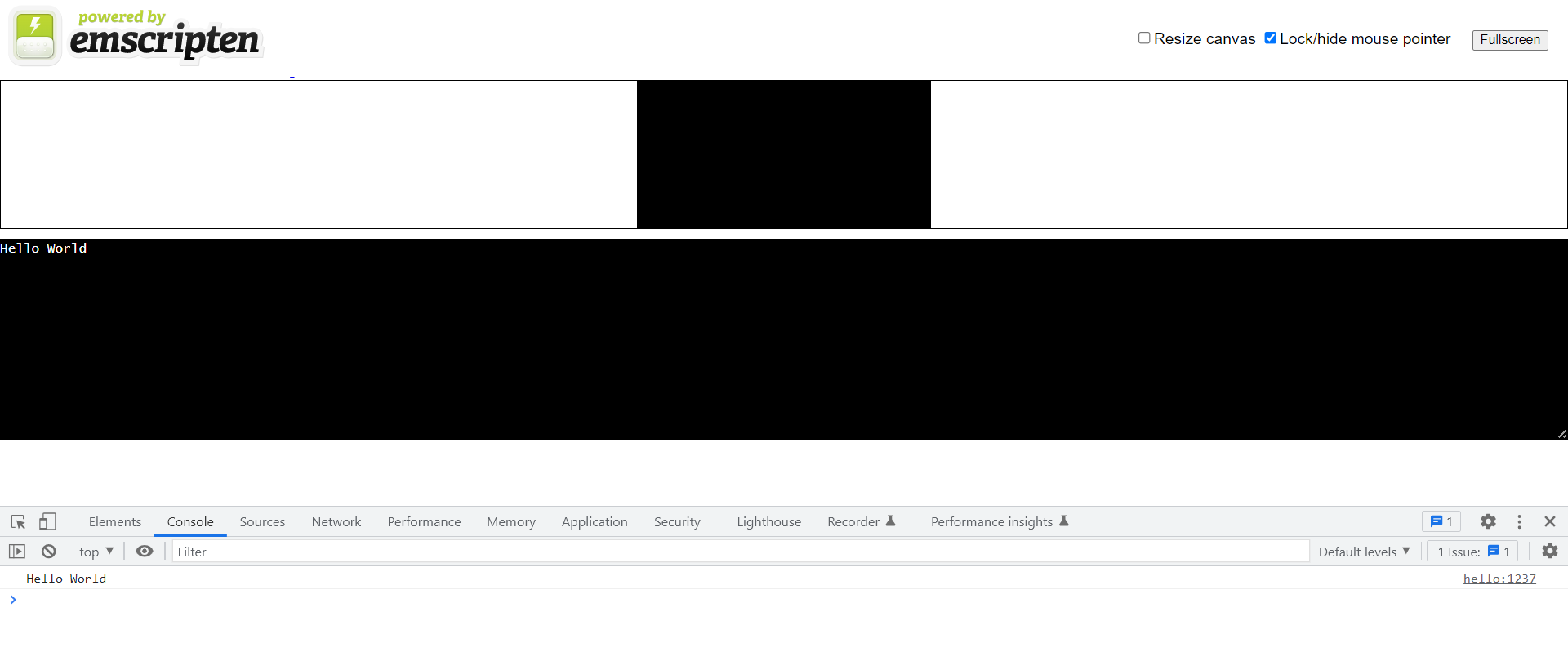``````#include <stdio.h>
#include <emscripten/emscripten.h>

int main() {
printf("Hello World\n");
return 0;
}

#ifdef __cplusplus
#define EXTERN extern "C"
#else
#define EXTERN
#endif

EXTERN EMSCRIPTEN_KEEPALIVE void greet(char* name) {
printf("Hello, %s!\n", name);
}``````

``\$ emcc -o greet.html greet.c -s NO_EXIT_RUNTIME=1 -s EXPORTED_RUNTIME_METHODS=ccall``

greet.html 文件和上面的 hello.html 几乎是一样的，我们在该文件中加几行代码来测试我们的 `greet()` 函数，首先加一个按钮：

``<button id="mybutton">Click me!</button>``

``````document.getElementById("mybutton").addEventListener("click", () => {
const result = Module.ccall(
"greet",         // name of C function
null,            // return type
["string"],      // argument types
["WebAssembly"]  // arguments
);
});``````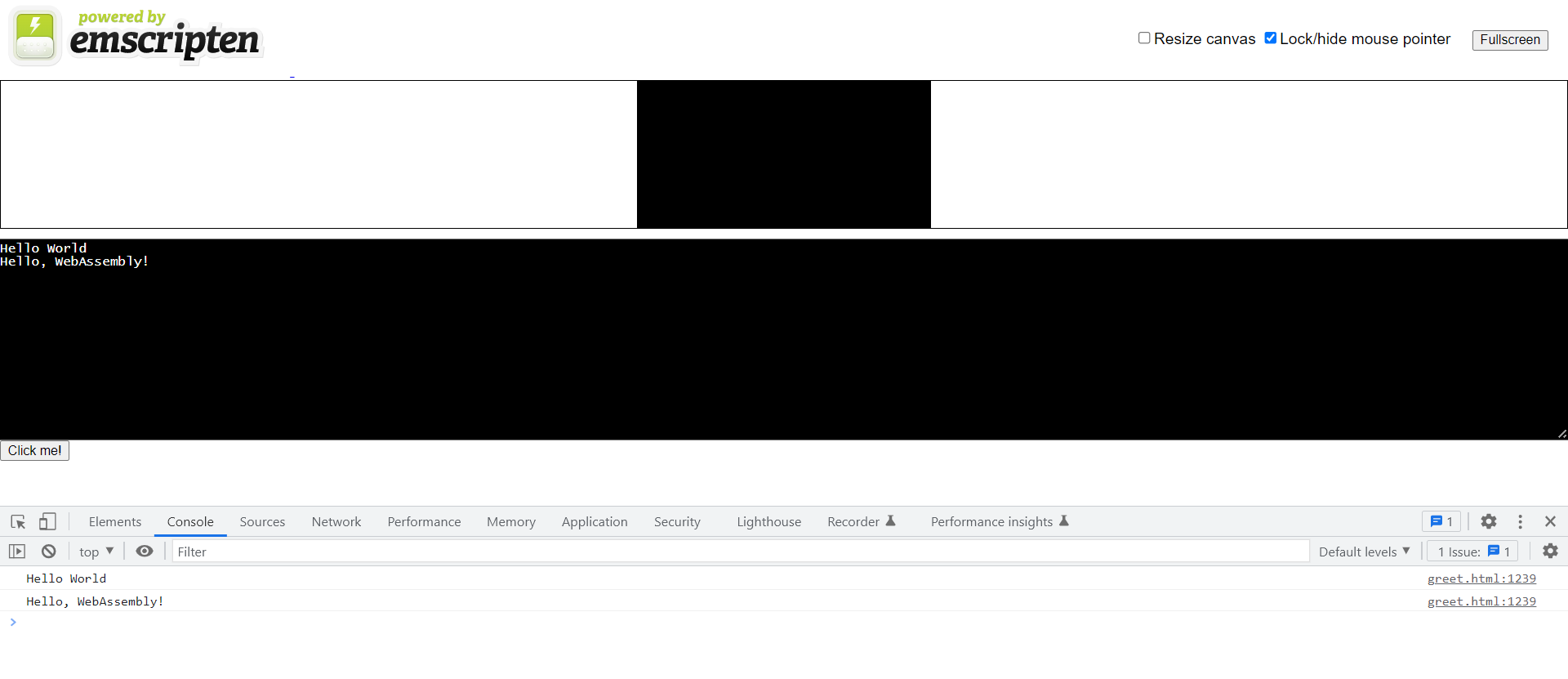### 将 Rust 程序编译成 WebAssembly

``\$ cargo install wasm-pack``

`wasm-pack` 用于将 Rust 代码编译成 WebAssembly 格式，不过要注意它不支持 bin 项目，只支持 lib 项目，所以我们通过 `--lib` 来创建项目：

``````\$ cargo new --lib rust-demo
Created library `rust-demo` package``````

``````use wasm_bindgen::prelude::*;

#[wasm_bindgen]
extern {
}

#[wasm_bindgen]
pub fn greet(name: &str) {
}``````

``````[lib]
crate-type = ["cdylib"]

[dependencies]
wasm-bindgen = "0.2"``````

``\$ wasm-pack build --target web``

``````<!DOCTYPE html>
<html lang="en-US">
<meta charset="utf-8" />
<title>hello-wasm example</title>
<body>
<script type="module">
import init, { greet } from "./pkg/rust_demo.js";
init().then(() => {
greet("WebAssembly");
});
</script>
</body>
</html>``````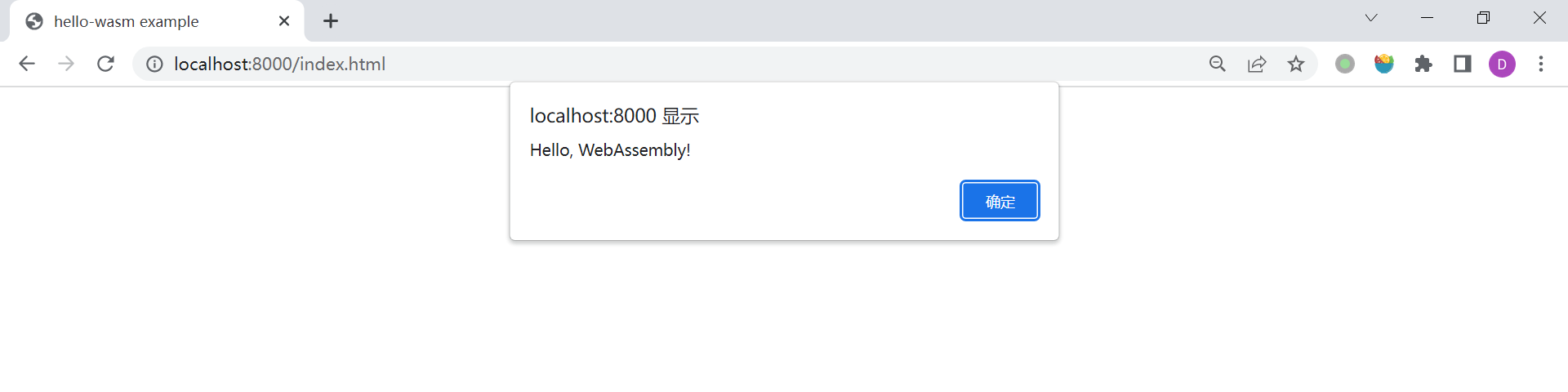### 将 Go 程序编译成 WebAssembly

``````\$ go version
go version go1.19 linux/amd64``````

``````\$ mkdir go-demo && cd go-demo
\$ go mod init com.example``````

``````package main

import "fmt"

func main() {
fmt.Println("Hello, WebAssembly!")
}``````

``\$ GOOS=js GOARCH=wasm go build -o main.wasm``

``\$ cp "\$(go env GOROOT)/misc/wasm/wasm_exec.js" .``

``````<html>
<meta charset="utf-8"/>
<script src="wasm_exec.js"></script>
<script>
const go = new Go();
WebAssembly.instantiateStreaming(fetch("main.wasm"), go.importObject).then((result) => {
go.run(result.instance);
});
</script>
<body></body>
</html>``````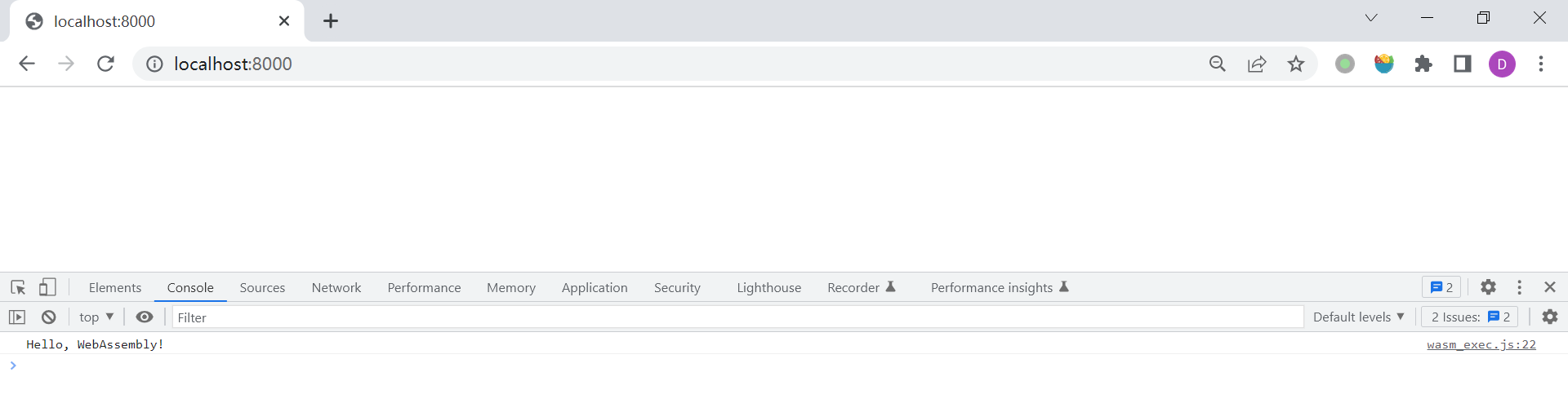``````\$ \$(go env GOROOT)/misc/wasm/go_js_wasm_exec ./main.wasm
Hello, WebAssembly!``````

``````\$ GOOS=js GOARCH=wasm go run -exec="\$(go env GOROOT)/misc/wasm/go_js_wasm_exec" .
Hello, WebAssembly!``````

``````package main

import (
"syscall/js"
)

func addFunction(this js.Value, p []js.Value) interface{} {
sum := p.Int() + p.Int()
return js.ValueOf(sum)
}

func main() {
select {} // block the main thread forever
}``````

``````wasm_exec.js:536 Uncaught Error: Go program has already exited
at globalThis.Go._resume (wasm_exec.js:536:11)
at wasm_exec.js:549:8
at <anonymous>:1:1``````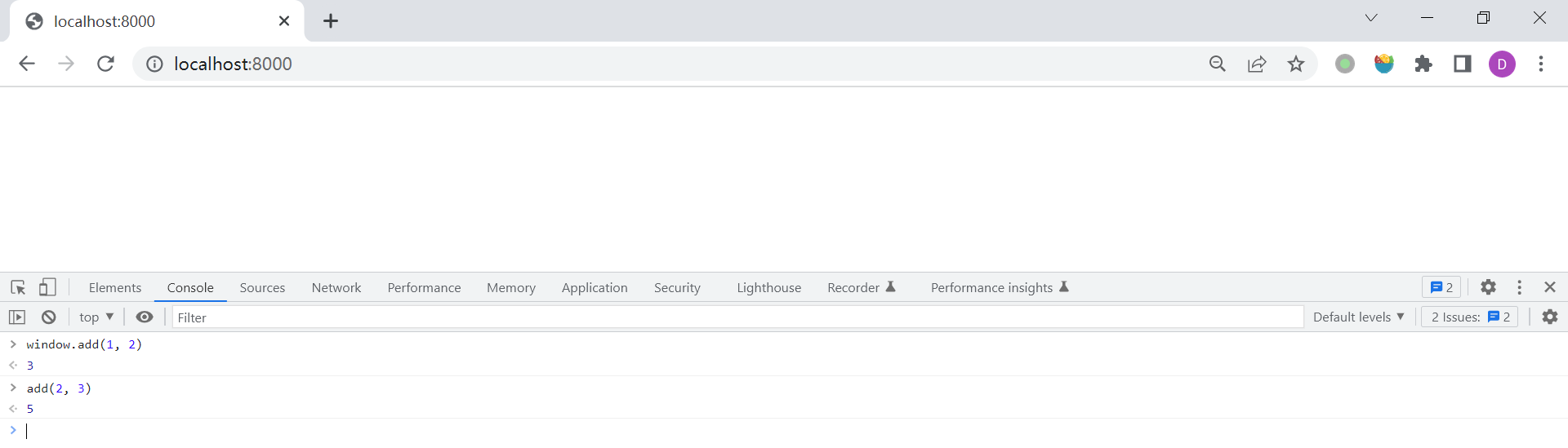`js.Global()` 为我们提供了一个 Go 和 JavaScript 之间的桥梁，我们不仅可以将 Go 函数暴露给 JavaScript 调用，甚至可以通过 `js.Global()` 来操作 DOM：

``````func hello(this js.Value, args []js.Value) interface{} {
doc := js.Global().Get("document")
h1 := doc.Call("createElement", "h1")
h1.Set("innerText", "Hello World")
doc.Get("body").Call("append", h1)
return nil
}``````

## WebAssembly 文本格式

``````(module
(func \$add (param \$lhs i32) (param \$rhs i32) (result i32)
local.get \$lhs
local.get \$rhs
)``````

WebAssembly 代码中的基本单元是一个模块，每个模块通过一个大的 S-表达式 来表示，S-表达式是一种嵌套结构，实际上它是树的一种表示形式。上面的代码首先通过 `(module)` 定义了一个模块，然后模块中使用 `(func \$add (param \$lhs i32) (param \$rhs i32) (result i32))` 定义了一个 `add()` 函数，这个 S-表达式转换为比较好理解的形式就是 `i32 add(i32 lhs, i32 rhs)`，最后通过 `(export "add" (func \$add))` 将该函数暴露出来，关于这段代码的详细解释可以参考 Mozilla 官方文档中的 Understanding WebAssembly text format

``\$ wat2wasm add.wat -o add.wasm``

``````fetchAndInstantiate('add.wasm').then(function(instance) {
});

// fetchAndInstantiate() found in wasm-utils.js
function fetchAndInstantiate(url, importObject) {
return fetch(url).then(response =>
response.arrayBuffer()
).then(bytes =>
WebAssembly.instantiate(bytes, importObject)
).then(results =>
results.instance
);
}``````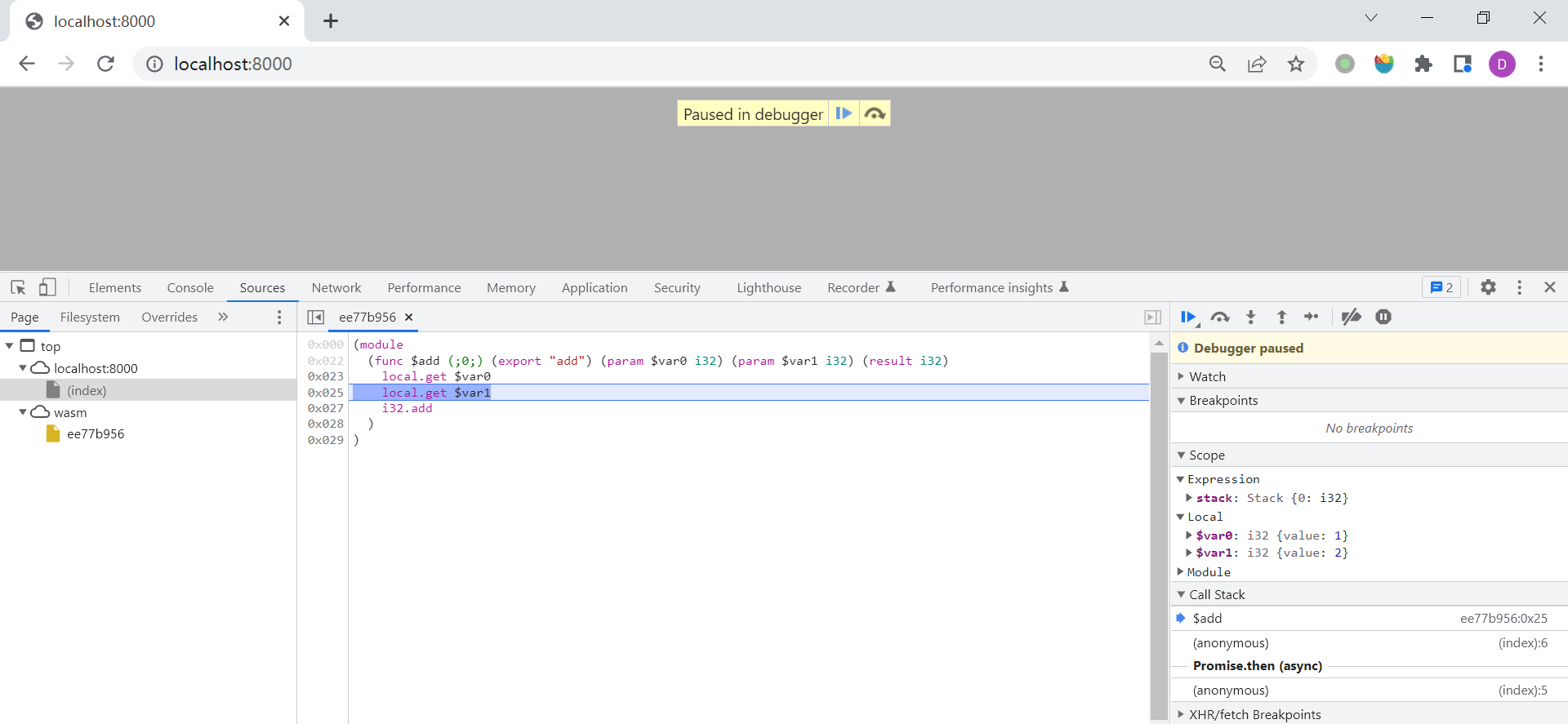## 更多

### 在非浏览器下运行 WebAssembly

WebAssembly 最早只应用于 Web 浏览器中，但鉴于它所拥有 可移植、安全及高效 等特性，WebAssembly 也被逐渐应用在 Web 领域之外的一些其他场景中，并为此提出了一项新的接口标准 —— WASI（WebAssembly System Interface）

### 使用 WABT 工具包

WABT 工具包中除了上文所使用的 `wat2wasm` 之外，还提供了很多其他有用的工具：

## Java 8 之 Stream API 用法总结

Java 编程语言发展迅速，从 Java 9 开始，Java 采取了小步迭代的发布方式，以每 6 个月发布一个版本的速度在持续更新，目前最新的版本已经升到 19 了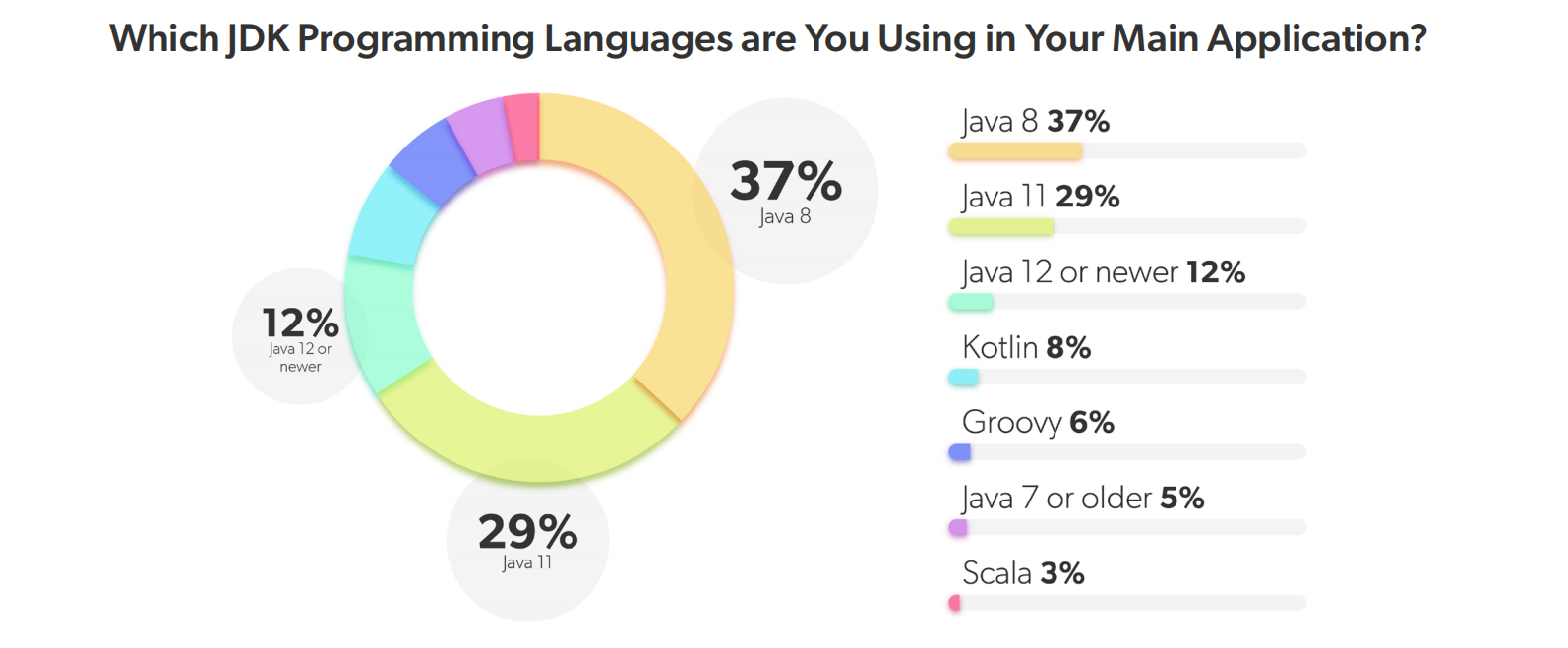Java 8 由 Oracle 公司于 2014 年 3 月 18 日发布，在这个版本中新增了大量的特性，首次引入了 Lambda 表达式和方法引用，开启了 Java 语言函数式编程的大门，其中新增的 Stream API（`java.util.stream`）特性更是将函数式编程发挥到了淋漓尽致的地步。

## Stream API 概述

``````List<String> words = List.of("A", "B", "C");
for (String word: words) {
System.out.println(word.toLowerCase());
}``````

``````List<String> words = List.of("A", "B", "C");
Iterator<String> iterator = words.iterator();
while (iterator.hasNext()) {
System.out.println(iterator.next().toLowerCase());
}``````

``````List<String> words = List.of("A", "B", "C");
words.stream().forEach(word -> System.out.println(word.toLowerCase()));``````

``````List<String> names = students.stream()
.filter(s -> s.getScore() >= 60)
.sorted((x, y) -> x.getScore() - y.getScore())
.map(Student::getName)
.collect(Collectors.toList());``````

## Stream API 使用

### 流的创建

JDK 中提供了很多途径来创建一个流，这一节总结一些常用的创建流的方法。流有一个很重要的特性：不会对数据源进行修改，所以我们可以对同一个数据源创建多个流。

#### 创建一个空流

``Stream<String> streamEmpty = Stream.empty();``

``````public Stream<String> streamOf(List<String> list) {
return list == null || list.isEmpty() ? Stream.empty() : list.stream();
}``````

#### 从集合类创建流

JDK 中自带了大量的集合类，比如 `List``Set``Queue` 以及它们的子类，这些类都继承自 `Collection` 接口：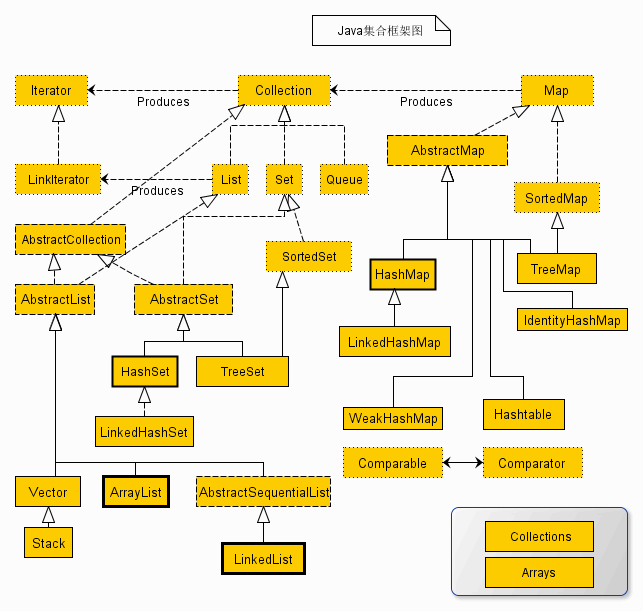``````List<String> collection = Arrays.asList("a", "b", "c");
Stream<String> streamOfCollection = collection.stream();``````

#### 从数组创建流

``````String[] array = new String[]{"a", "b", "c"};
Stream<String> streamOfArray = Arrays.stream(array);``````

``Stream<String> streamOfArray2 = Stream.of(array);``

``Stream<String> streamOfArray3 = Stream.of("a", "b", "c");``

#### 使用 `Stream.builder()` 手工创建流

``````Stream<String> streamOfBuilder = Stream.<String>builder()
.build();``````

``````Stream.Builder<String> builder = Stream.<String>builder();
Stream<String> streamOfBuilder2 = builder.build();``````

#### 使用 `Stream.generate()` 生成流

`Stream.generate()` 方法也可以用于手工创建流，这个方法需要提供一个 `Supplier<T>` 的实现，生成的是一个无限流，一般通过 `limit` 来限定数量：

``Stream<String> streamOfGenerate = Stream.generate(() -> "hello").limit(3);``

``````AtomicInteger num = new AtomicInteger(0);
Stream<Integer> streamOfGenerate2 = Stream.generate(() -> num.incrementAndGet()).limit(3);``````

#### 使用 `Stream.iterate()` 生成流

``Stream<Integer> streamOfIterate = Stream.iterate(1, n -> n + 1).limit(3);``

`iterate()` 函数第一个参数为流的第一个元素，后续的元素通过第二个参数中的 `UnaryOperator<T>` 来迭代生成。

#### 生成基础类型的流

``IntStream intStream = IntStream.range(1, 4);``

``IntStream intStream2 = IntStream.rangeClosed(1, 3);``

``IntStream intStream = new Random().ints(3);``

``````LongStream longStream = new Random().longs(3);
DoubleStream doubleStream = new Random().doubles(3);``````

#### 使用 `String.chars()` 生成字符流

`String` 类提供了一个 `chars()` 方法，用于从字符串生成字符流，正如上面所说，JDK 只提供了 `IntStream``LongStream``DoubleStream` 三种基础类型流，并没有 `CharStream` 一说，所以返回值使用了 `IntStream`

``IntStream charStream = "abc".chars();``

#### 使用 `Pattern.splitAsStream()` 生成字符串流

``Stream<String> stringStream = Pattern.compile(", ").splitAsStream("a, b, c");``

#### 从文件生成字符串流

``````try (Stream<String> stringStream = Files.lines(Paths.get(filePath + "test.txt"));) {
stringStream.forEach(System.out::println);
}``````

### 中间操作

``````Stream<Student> students = Stream.of(
Student.builder().name("张三").gender("男").age(27).number(3L).interests("画画、篮球").build(),
Student.builder().name("李四").gender("男").age(29).number(2L).interests("篮球、足球").build(),
Student.builder().name("王二").gender("女").age(27).number(1L).interests("唱歌、跳舞、画画").build(),
Student.builder().name("麻子").gender("女").age(31).number(4L).interests("篮球、羽毛球").build()
);``````

#### 无状态操作

##### `filter`

`filter` 用于对数据流进行过滤，它接受一个 `Predicate<? super T> predicate` 参数，返回符合该 Predicate 条件的元素：

``students = students.filter(s -> s.getAge() > 30);``
##### `map` / `mapToInt` / `mapToLong` / `mapToDouble`

`map` 接受一个 `Function<? super T, ? extends R> mapper` 类型的参数，对数据流的类型进行转换，从 T 类型转换为 R 类型，比如下面的代码将数据流 `Stream<Student>` 转换为 `Stream<StudentDTO>`

``````Stream<StudentDTO> studentDTOs = students.map(s -> {
return StudentDTO.builder().name(s.getName()).age(s.getAge()).build();
});``````

``LongStream studentAges = students.mapToLong(s -> s.getAge());``

``LongStream studentAges2 = students.mapToLong(Student::getAge);``
##### `flatMap` / `flatMapToInt` / `flatMapToLong` / `flatMapToDouble`

`flatMap` 接受一个 `Function<? super T, ? extends Stream<? extends R>> mapper` 类型的参数，和 `map` 不同的是，他将 T 类型转换为 R 类型的流，而不是转换为 R 类型，然后再将流中所有数据平铺得到最后的结果：

``Stream<String> studentInterests = students.flatMap(s -> Arrays.stream(s.getInterests().split("、")));``

##### `peek`

`peek` 一般用来调试，它接受一个 `Consumer<? super T> action` 参数，可以在流的计算过程中对元素进行处理，无返回结果，比如打印出元素的状态：

``````Stream<String> studentNames = students.filter(s -> s.getAge() > 20)
.peek(System.out::println)
.map(Student::getName)
.peek(System.out::println);``````
##### `unordered`

``````List<Integer> ints = Stream.of(1, 2, 3).unordered()
.map(x -> x*2)
.collect(Collectors.toList());``````

#### 有状态操作

##### `distinct`

`distinct()` 方法用于去除流中的重复元素：

``````Stream<Integer> intStream = Stream.of(1, 2, 3, 2, 4, 3, 1, 2);
intStream = intStream.distinct();``````

`distinct()` 是根据流中每个元素的 `equals()` 方法来去重的，所以如果流中是对象类型，可能需要重写其 `equals()` 方法。

##### `sorted`

`sorted()` 方法根据 自然序（natural order） 对流中的元素进行排序，流中的元素必须实现 `Comparable` 接口：

``````Stream<Integer> intStream = Stream.of(1, 3, 2, 4);
intStream = intStream.sorted();``````

``````students = students.sorted(new Comparator<Student>() {

@Override
public int compare(Student o1, Student o2) {
return o1.getAge().compareTo(o2.getAge());
}

});``````

``students = students.sorted((o1, o2) -> o1.getAge().compareTo(o2.getAge()));``

``students = students.sorted(Comparator.comparing(Student::getAge));``

``````students = students.sorted(
Comparator.comparing(Student::getAge).thenComparing(Student::getNumber)
);``````
##### `skip` / `limit`

`skip``limit` 这两个方法有点类似于 SQL 中的 `LIMIT offset, rows` 语句，用于返回指定的记录条数，最常见的一个用处是用来做分页查询。

``````Stream<Integer> intStream = Stream.of(1, 2, 3, 4, 5, 6, 7, 8, 9, 10);
intStream = intStream.skip(3).limit(3);``````
##### `dropWhile` / `takeWhile`

`dropWhile``takeWhile` 这两个方法的作用也是返回指定的记录条数，只不过条数不是固定的，而是根据某个条件来决定返回哪些元素：

``````Stream<Integer> intStream = Stream.of(1, 2, 3, 4, 5, 6, 7, 8, 9, 10);
intStream = intStream.dropWhile(x -> x <= 3).takeWhile(x -> x <= 6);``````

### 结束操作

#### 短路操作

##### `anyMatch` / `allMatch` / `nonMatch`

`anyMatch` 表示只要有一个元素满足条件即返回 `true`

``boolean hasAgeGreaterThan30 = students.anyMatch(s -> s.getAge() > 30);``

`allMatch` 表示所有元素都满足条件才返回 `true`

``boolean allAgeGreaterThan20 = students.allMatch(s -> s.getAge() > 20);``

`noneMatch` 表示所有元素都不满足条件才返回 `true`

``boolean noAgeGreaterThan40 = students.noneMatch(s -> s.getAge() > 40);``
##### `findFirst` / `findAny`

`findFirst` 用于返回流中第一个元素：

``````// 返回的是 李四
Optional<Student> student = students.filter(s -> s.getAge() > 28).findFirst();``````

`findAny()` 返回的元素是不确定的，如果是串行流，返回的是第一个元素：

``````// 返回的是 李四
Optional<Student> student = students.filter(s -> s.getAge() > 28).findAny();``````

``````// 返回不确定
Optional<Student> student = students.parallel().filter(s -> s.getAge() > 28).findAny();``````

#### 非短路操作

##### `forEach` / `forEachOrdered`

``intStream.forEach(System.out::println);``

``intStream.parallel().forEach(System.out::println);``

``intStream.parallel().forEachOrdered(System.out::println);``
##### `toArray`

`toArray` 方法用于将流转换为一个数组，默认情况下数组类型是 `Object[]`

``Object[] array = students.toArray();``

``Student[] array = students.toArray(Student[]::new);``
##### `reduce`

`reduce` 方法有三种不同的函数形式，第一种也是最简单的：

``Optional<T> reduce(BinaryOperator<T> accumulator);``

``````@FunctionalInterface
public interface BiFunction<T, U, R> {
// ...
}``````

`BinaryOperator` 同样也是两个入参和一个出参的函数，但是它的两个入参的类型和出参的类型是一样的：

``````@FunctionalInterface
public interface BinaryOperator<T> extends BiFunction<T,T,T> {
// ...
}``````

`accumulator` 的意思是累加器，它是一个函数，它有两个参数。它的第一个参数是上次函数执行的返回值（也称为中间结果），第二个参数是流中的元素，函数将两个值按照方法进行处理，得到值赋给下次执行这个函数的参数。第一次执行的时候第一参数的值是流中第一元素，第二个元素是流中第二元素，因为流可能为空，所以这个方法的返回值为 `Optional`

``Optional<Integer> result = students.map(Student::getAge).reduce((x, y) -> x + y);``

``Optional<Integer> result = students.map(Student::getAge).reduce(Integer::sum);``

``Optional<Integer> result = students.map(Student::getAge).reduce((x, y) -> x > y ? x : y);``

``Optional<Integer> result = students.map(Student::getAge).reduce((x, y) -> x < y ? x : y);``

`reduce` 的第二种形式是：

``T reduce(T identity, BinaryOperator<T> accumulator);``

``Integer result = students.map(Student::getAge).reduce(0, Integer::sum);``

`reduce` 的 JDK 源码注释里，有一段伪代码很好地解释了 `reduce` 内部的处理逻辑：

``````U result = identity;
for (T element : this stream)
result = accumulator.apply(result, element)
return result;``````

`reduce` 的第三种形式如下：

``````<U> U reduce(U identity,
BiFunction<U, ? super T, U> accumulator,
BinaryOperator<U> combiner);``````

``Integer result = intStream.parallel().reduce(0, Integer::sum);``

``Integer result = intStream.parallel().reduce(0, Integer::sum, Integer::sum);``

``````Stream<Integer> intStream = Stream.of(1, 3, 2, 4, 2, 4, 2);
Map<Integer, Integer> countMap = intStream.reduce(new HashMap<>(), (x, y) -> {
if (x.containsKey(y)) {
x.put(y, x.get(y) + 1);
} else {
x.put(y, 1);
}
return x;
}, (x, y) -> new HashMap<>());``````

``````Stream<Integer> intStream = Stream.of(1, 3, 2, 4, 2, 4, 2);
List<Integer> distinctMap = intStream.reduce(new ArrayList<>(), (x, y) -> {
if (!x.contains(y)) {
}
return x;
}, (x, y) -> new ArrayList<>());``````

List 转 Map：

``````Map<Long, Student> studentMap = students.reduce(new HashMap<Long, Student>(), (x, y) -> {
x.put(y.getNumber(), y);
return x;
}, (x, y) -> new HashMap<Long, Student>());``````

``````Map<Long, Student> studentMap = students.parallel().reduce(new HashMap<Long, Student>(), (x, y) -> {
x.put(y.getNumber(), y);
return x;
}, (x, y) -> {
for (Map.Entry<Long, Student> entry : y.entrySet()) {
x.put(entry.getKey(), entry.getValue());
}
return x;
});``````
##### `collect`

`collect` 函数正如它的名字一样，可以将流中的元素经过处理并收集起来，得到收集后的结果，这听起来感觉和 `reduce` 函数有点像，而且它的函数定义也和 `reduce` 函数很类似：

``````<R> R collect(Supplier<R> supplier,
BiConsumer<R, ? super T> accumulator,
BiConsumer<R, R> combiner);``````

``````Stream<Integer> intStream = Stream.of(1, 3, 2, 4);
AtomicInteger result = intStream.collect(
() -> new AtomicInteger(),
(a, b) -> {}
);``````

``<R, A> R collect(Collector<? super T, A, R> collector);``

``List<Integer> result = intStream.collect(Collectors.toList());``

``````public static <T>
Collector<T, ?, List<T>> toList() {
return new CollectorImpl<>(
(Supplier<List<T>>) ArrayList::new,
(left, right) -> { left.addAll(right); return left; },
CH_ID);
}``````

``Integer result = intStream.collect(Collectors.summingInt(Integer::valueOf));``

``Optional<Integer> result = intStream.collect(Collectors.maxBy(Integer::compareTo));``

``Map<Integer, Long> result = intStream.collect(Collectors.groupingBy(i -> i, Collectors.counting()));``

``Map<Integer, Integer> result = intStream.collect(Collectors.toMap(i -> i, i -> i, (i, j) -> i));``

List 转 Map：

``Map<Long, Student> result = students.collect(Collectors.toMap(Student::getNumber, Function.identity()));``

``String result = students.map(Student::getName).collect(Collectors.joining("、"));``

``Map<Boolean, List<Student>> result = students.collect(Collectors.partitioningBy(x -> x.getAge() > 30));``

``Map<Integer, List<Student>> result = students.collect(Collectors.groupingBy(Student::getAge));``

`partitioningBy``groupingBy` 函数非常类似，只不过一个将数据分成两组，一个将数据分为多组，它们的第一个参数都是 `Function<? super T, ? extends K> classifier`，又被称为 分类函数（classification function），分组返回的 `Map` 的键就是由它产生的，而对应的 `Map` 的值是该分类的数据列表。很容易想到，既然得到了每个分类的数据列表，我们当然可以继续使用 Stream API 对每个分类的数据进一步处理。所以 `groupingBy` 函数还提供了另一种形式：

``````Collector<T, ?, Map<K, D>> groupingBy(
Function<? super T, ? extends K> classifier,
Collector<? super T, A, D> downstream)``````

``Map<Integer, Long> result = intStream.collect(Collectors.groupingBy(i -> i, Collectors.counting()));``

``````Map<String, List<String>> result = students.collect(Collectors.groupingBy(
Student::getGender, Collectors.mapping(
Student::getName, Collectors.toList())));``````

#### `count`

`count` 比较简单，用于统计流中元素个数：

``long count = students.count();``
##### `max` / `min`

`max``min` 函数用于计算流中的最大元素和最小元素，元素的大小通过比较器 `Comparator<? super T> comparator` 来决定。比如获取年龄最大的学生：

``Optional<Student> maxAgeStudent = students.max(Comparator.comparingInt(Student::getAge));``

``OptionalInt maxAge = students.mapToInt(Student::getAge).max();``
##### `sum` / `average` / `summaryStatistics`

``int sumAge = students.mapToInt(Student::getAge).sum();``

`average` 用于求平均值：

``OptionalDouble averageAge = students.mapToInt(Student::getAge).average();``

`summaryStatistics` 用于一次性获取流中数据的统计信息（包括最大值、最小值、总和、数量、平均值）：

``````IntSummaryStatistics summaryStatistics = students.mapToInt(Student::getAge).summaryStatistics();
System.out.println("Max = " + summaryStatistics.getMax());
System.out.println("Min = " + summaryStatistics.getMin());
System.out.println("Sum = " + summaryStatistics.getSum());
System.out.println("Count = " + summaryStatistics.getCount());
System.out.println("Average = " + summaryStatistics.getAverage());``````

## 更多

### `Collectors` 静态方法一览

• 转换为集合

• `Collector<T, ?, C> toCollection(Supplier<C> collectionFactory)`
• `Collector<T, ?, List<T>> toList()`
• `Collector<T, ?, Set<T>> toSet()`
• 统计计算

• `Collector<T, ?, IntSummaryStatistics> summarizingInt(ToIntFunction<? super T> mapper)`
• `Collector<T, ?, LongSummaryStatistics> summarizingLong(ToLongFunction<? super T> mapper)`
• `Collector<T, ?, DoubleSummaryStatistics> summarizingDouble(ToDoubleFunction<? super T> mapper)`
• `Collector<T, ?, Optional<T>> minBy(Comparator<? super T> comparator)`
• `Collector<T, ?, Optional<T>> maxBy(Comparator<? super T> comparator)`
• `Collector<T, ?, Integer> summingInt(ToIntFunction<? super T> mapper)`
• `Collector<T, ?, Long> summingLong(ToLongFunction<? super T> mapper)`
• `Collector<T, ?, Double> summingDouble(ToDoubleFunction<? super T> mapper)`
• `Collector<T, ?, Double> averagingInt(ToIntFunction<? super T> mapper)`
• `Collector<T, ?, Double> averagingLong(ToLongFunction<? super T> mapper)`
• `Collector<T, ?, Double> averagingDouble(ToDoubleFunction<? super T> mapper)`
• `Collector<T, ?, Long> counting()`
• 字符串拼接

• `Collector<CharSequence, ?, String> joining()`
• `Collector<CharSequence, ?, String> joining(CharSequence delimiter)`
• `Collector<CharSequence, ?, String> joining(CharSequence delimiter, CharSequence prefix, CharSequence suffix)`
• Map & Reduce

• `Collector<T, ?, R> mapping(Function<? super T, ? extends U> mapper, Collector<? super U, A, R> downstream)`
• `Collector<T, ?, T> reducing(T identity, BinaryOperator<T> op)`
• `Collector<T, ?, Optional<T>> reducing(BinaryOperator<T> op)`
• `Collector<T, ?, U> reducing(U identity, Function<? super T, ? extends U> mapper, BinaryOperator<U> op)`
• 分组

• `Collector<T, ?, Map<K, List<T>>> groupingBy(Function<? super T, ? extends K> classifier)`
• `Collector<T, ?, Map<K, D>> groupingBy(Function<? super T, ? extends K> classifier, Collector<? super T, A, D> downstream)`
• `Collector<T, ?, M> groupingBy(Function<? super T, ? extends K> classifier, Supplier<M> mapFactory, Collector<? super T, A, D> downstream)`
• `Collector<T, ?, ConcurrentMap<K, List<T>>> groupingByConcurrent(Function<? super T, ? extends K> classifier)`
• `Collector<T, ?, ConcurrentMap<K, D>> groupingByConcurrent(Function<? super T, ? extends K> classifier, Collector<? super T, A, D> downstream)`
• `Collector<T, ?, M> groupingByConcurrent(Function<? super T, ? extends K> classifier, Supplier<M> mapFactory, Collector<? super T, A, D> downstream)`
• `Collector<T, ?, Map<Boolean, List<T>>> partitioningBy(Predicate<? super T> predicate)`
• `Collector<T, ?, Map<Boolean, D>> partitioningBy(Predicate<? super T> predicate, Collector<? super T, A, D> downstream)`
• List 转 Map

• `Collector<T, ?, Map<K,U>> toMap(Function<? super T, ? extends K> keyMapper, Function<? super T, ? extends U> valueMapper)`
• `Collector<T, ?, Map<K,U>> toMap(Function<? super T, ? extends K> keyMapper, Function<? super T, ? extends U> valueMapper, BinaryOperator<U> mergeFunction)`
• `Collector<T, ?, M> toMap(Function<? super T, ? extends K> keyMapper, Function<? super T, ? extends U> valueMapper, BinaryOperator<U> mergeFunction, Supplier<M> mapSupplier)`
• `Collector<T, ?, ConcurrentMap<K,U>> toConcurrentMap(Function<? super T, ? extends K> keyMapper, Function<? super T, ? extends U> valueMapper)`
• `Collector<T, ?, ConcurrentMap<K,U>> toConcurrentMap(Function<? super T, ? extends K> keyMapper, Function<? super T, ? extends U> valueMapper, BinaryOperator<U> mergeFunction)`
• `Collector<T, ?, M> toConcurrentMap(Function<? super T, ? extends K> keyMapper, Function<? super T, ? extends U> valueMapper, BinaryOperator<U> mergeFunction, Supplier<M> mapSupplier)`
• 其他

• `Collector<T,A,RR> collectingAndThen(Collector<T,A,R> downstream, Function<R,RR> finisher)`